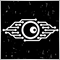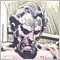# the condition is not met, how to break19130391

Esse:
Kicking it hard...4045

Minions Labs:
Kicking it hard...

And if that doesn't work, kick it again.

Look here: https://docs.mql4.com/basis/operators/if19

Anthony Garot:

And if that doesn't work, kick it again.

Look here: https://docs.mql4.com/basis/operators/if

it was like that:

```for(x=0; x<201; x++){
if(
Open(5+x) < Close (5+x) &&
Open(4+x) < Close (4+x) &&
Open(3+x) < Close (3+x) &&
Open(2+x) < Close (2+x) )
}```

I changed to:

```for(x=0; x<201; x++){

if (Open(5+x) < Close (5+x)) continue; else break;
if (Open(4+x) < Close (4+x)) continue; else break;
if (Open(3+x) < Close (3+x)) continue; else break;
if (Open(2+x) < Close (2+x)) continue; else break;
}
nr_line=x```

It's ok now?4045

Esse:

It's ok now?

Not exactly sure what you are trying to do here, but you could simplify your code by doing the following:

```for(x=0; x<201; x++)
{
if (Open(5+x) >= Close(5+x)) break;
if (Open(4+x) >= Close(4+x)) break;
if (Open(3+x) >= Close(3+x)) break;
if (Open(2+x) >= Close(2+x)) break;
}```19

Anthony Garot:

Not exactly sure what you are trying to do here, but you could simplify your code by doing the following:

Thanks, it's works!!

The code has accelerated - 17 times faster:

8 minut 25 sekund ===> now: 30 sekund